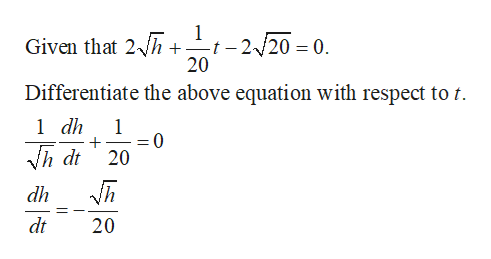# Water flows from a tank of constant cross-sectional area 40ft^2 through an orifice of constant cross-sectional area 1/4ft^2 located at the bottom of the tank. Initially, the height of the water in the tank was 20ft, and t sec later it was given by the equation2(h^1/2) + (1/20)t - 2(20^1/2) = 0         0<= t <= 40(20^1/2)How fast was the height of the water decreasing when its height was 16ft?

Question
11 views

Water flows from a tank of constant cross-sectional area 40ft^2 through an orifice of constant cross-sectional area 1/4ft^2 located at the bottom of the tank. Initially, the height of the water in the tank was 20ft, and t sec later it was given by the equation

2(h^1/2) + (1/20)t - 2(20^1/2) = 0         0<= t <= 40(20^1/2)

How fast was the height of the water decreasing when its height was 16ft?

check_circle

Step 1

Compute the der...help_outlineImage Transcriptionclose1 -t - 2/20 = 0. 20 Given that 2h Differentiate the above equation with respect to t. 1 dh 1 0 20 dh dt 20 fullscreen

### Want to see the full answer?

See Solution

#### Want to see this answer and more?

Solutions are written by subject experts who are available 24/7. Questions are typically answered within 1 hour.*

See Solution
*Response times may vary by subject and question.
Tagged in

### Other